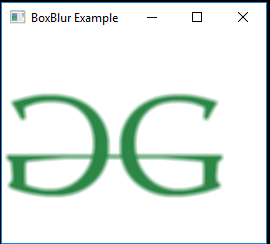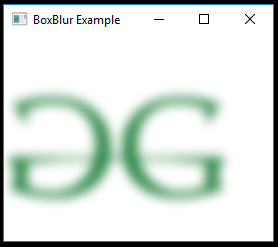# JavaFX | BoxBlur Class

BoxBlur class is part of JavaFX. BoxBlur uses a simple Box filter to blur a node. The BoxBlur is used to implement Blur in JavaFX. A blur effect using a simple box filter kernel, with separately configurable sizes in both dimensions, and an iteration parameter that controls the quality of the resulting blur.

The BoxBlur class has three components:

1. Height: The vertical dimension of the effect.
2. Width: The horizontal dimension of the effect.
3. Iterations: The number of iterations of the blur effect.

Constructors of the class:

1. BoxBlur(): Creates a new object of BoxBlur.
2. BoxBlur(double w, double h, int i): Creates a new BoxBlur object with specified width, height and iterations.

Commonly Used Methods:

Method Explanation
getHeight() Returns the vertical dimension of the effect
getWidth() Returns the horizontal dimension of the effect
getIterations() Returns the number of iterations of the effect
getInput() Gets the value of the property input.
setInput(Effect v) Sets the value of the property input.
setHeight(double v) Sets the vertical dimension of the effect
setWidth(double v) Sets the horizontal dimension of the effect
setIterations(int i) Sets the number of iterations of the effect

Below programs illustrate the use of BoxBlur class:

1. Java program to import an image and add Box Blur effect to it: In this program a FileInputStream is created and an image is taken as input from a file. Image named image is created using the input from the file input stream. From the image, an image view object is created and it is added to the VBox. The VBox is then added to the scene and the scene is added to the stage. A BoxBlur effect is created with a specified level passed as parameters and the effect is set to the image view using setEffect() function.

 `// Java program to import an image and  ` `// add Box Blur effect to it ` `import` `javafx.application.Application; ` `import` `javafx.scene.Scene; ` `import` `javafx.scene.control.*; ` `import` `javafx.scene.layout.*; ` `import` `javafx.stage.Stage; ` `import` `javafx.scene.image.*; ` `import` `javafx.scene.effect.*; ` `import` `java.io.*; ` `import` `javafx.event.ActionEvent; ` `import` `javafx.event.EventHandler; ` `import` `javafx.scene.Group; ` ` `  `public` `class` `box_blur_1 ``extends` `Application { ` ` `  `    ``// launch the application ` `    ``public` `void` `start(Stage stage) ``throws` `Exception ` `    ``{ ` ` `  `        ``// set title for the stage ` `        ``stage.setTitle(``"BoxBlur Example"``); ` ` `  `        ``// create a input stream ` `        ``FileInputStream input = ``new` `FileInputStream(``"D:\\GFG.png"``); ` ` `  `        ``// create a image ` `        ``Image image = ``new` `Image(input); ` ` `  `        ``// create a image View ` `        ``ImageView imageview = ``new` `ImageView(image); ` ` `  `        ``// create a box blur effect ` `        ``BoxBlur box_blur = ``new` `BoxBlur(); ` ` `  `        ``// set effect ` `        ``imageview.setEffect(box_blur); ` ` `  `        ``// create a VBox ` `        ``VBox vbox = ``new` `VBox(imageview); ` ` `  `        ``// create a scene ` `        ``Scene scene = ``new` `Scene(vbox, ``200``, ``200``); ` ` `  `        ``// set the scene ` `        ``stage.setScene(scene); ` ` `  `        ``stage.show(); ` `    ``} ` ` `  `    ``// Main Method ` `    ``public` `static` `void` `main(String args[]) ` `    ``{ ` ` `  `        ``// launch the application ` `        ``launch(args); ` `    ``} ` `} `

Input Image:Output:2. Java program to import an image and blur effect to it with separately configurable sizes in both dimensions, and an iteration parameter: In this program a FileInputStream is created and an image is taken as input from a file. Image named image is created using the input from file input stream. From the image, an image view object is created and it is added to the VBox. The VBox is then added to the scene and the scene is added to the stage. A BoxBlur effect is created with a specified level passed as parameters and the effect is set to the image view using setEffect() function. The width, height and the number of iterations of the effect is set using setHeight(), setWidth() and setIterations() function respectively.

 `// Java program to import an image and blur effect  ` `// to it with separately configurable sizes in both ` `// dimensions, and an iteration parameter ` `import` `javafx.application.Application; ` `import` `javafx.scene.Scene; ` `import` `javafx.scene.control.*; ` `import` `javafx.scene.layout.*; ` `import` `javafx.stage.Stage; ` `import` `javafx.scene.image.*; ` `import` `javafx.scene.effect.*; ` `import` `java.io.*; ` `import` `javafx.event.ActionEvent; ` `import` `javafx.event.EventHandler; ` `import` `javafx.scene.Group; ` ` `  `public` `class` `box_blur_2 ``extends` `Application { ` ` `  `    ``// launch the application ` `    ``public` `void` `start(Stage stage) ``throws` `Exception ` `    ``{ ` ` `  `        ``// set title for the stage ` `        ``stage.setTitle(``"BoxBlur Example"``); ` ` `  `        ``// create a input stream ` `        ``FileInputStream input = ``new` `FileInputStream(``"D:\\GFG.png"``); ` ` `  `        ``// create a image ` `        ``Image image = ``new` `Image(input); ` ` `  `        ``// create a image View ` `        ``ImageView imageview = ``new` `ImageView(image); ` ` `  `        ``// create a box blur effect ` `        ``BoxBlur box_blur = ``new` `BoxBlur(); ` ` `  `        ``// set width ` `        ``box_blur.setWidth(``10``.0f); ` ` `  `        ``// set height ` `        ``box_blur.setHeight(``10``.0f); ` ` `  `        ``// set Iterations ` `        ``box_blur.setIterations(``3``); ` ` `  `        ``// set effect ` `        ``imageview.setEffect(box_blur); ` ` `  `        ``// create a VBox ` `        ``VBox vbox = ``new` `VBox(imageview); ` ` `  `        ``// create a scene ` `        ``Scene scene = ``new` `Scene(vbox, ``200``, ``200``); ` ` `  `        ``// set the scene ` `        ``stage.setScene(scene); ` ` `  `        ``stage.show(); ` `    ``} ` ` `  `    ``// Main Method ` `    ``public` `static` `void` `main(String args[]) ` `    ``{ ` ` `  `        ``// launch the application ` `        ``launch(args); ` `    ``} ` `} `

Input Image:Output:Note: The above programs might not run in an online IDE. Please use an offline compiler.

My Personal Notes arrow_drop_upSecond year Department of Information Technology Jadavpur University

If you like GeeksforGeeks and would like to contribute, you can also write an article using contribute.geeksforgeeks.org or mail your article to contribute@geeksforgeeks.org. See your article appearing on the GeeksforGeeks main page and help other Geeks.

Please Improve this article if you find anything incorrect by clicking on the "Improve Article" button below.

Article Tags :
Practice Tags :

Be the First to upvote.

Please write to us at contribute@geeksforgeeks.org to report any issue with the above content.